# dwt2

Single-level 2-D discrete wavelet transform

## Syntax

``[cA,cH,cV,cD] = dwt2(X,wname)``
``[cA,cH,cV,cD] = dwt2(X,LoD,HiD)``
``[cA,cH,cV,cD] = dwt2(___,'mode',extmode)``

## Description

`dwt2` computes the single-level 2-D wavelet decomposition. Compare `dwt2` with `wavedec2` which may be more useful for your application. The decomposition is done with respect to either a particular wavelet (see `wfilters` for more information) or particular wavelet decomposition filters.

example

````[cA,cH,cV,cD] = dwt2(X,wname)` computes the single-level 2-D discrete wavelet transform (DWT) of the input data `X` using the `wname` wavelet. `dwt2` returns the approximation coefficients matrix `cA` and detail coefficients matrices `cH`, `cV`, and `cD` (horizontal, vertical, and diagonal, respectively).```

example

````[cA,cH,cV,cD] = dwt2(X,LoD,HiD)` computes the single-level 2-D DWT using the wavelet decomposition lowpass filter `LoD` and highpass filter `HiD`. The decomposition filters must have the same length and an even number of samples.```
````[cA,cH,cV,cD] = dwt2(___,'mode',extmode)` computes the single-level 2-D DWT with the extension mode `extmode`. Include this argument after all other arguments. NoteFor `gpuArray` inputs, the supported modes are `'symh'` (`'sym'`) and `'per'`. All `'mode'` options except `'per'` are converted to `'symh'`. See the example Single-Level 2-D Discrete Wavelet Transform on a GPU. ```

## Examples

collapse all

```load woman imagesc(X) colormap(map)```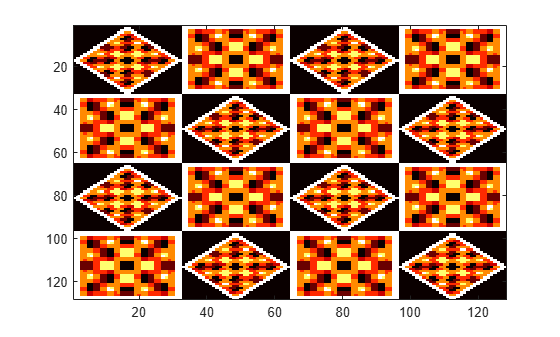Obtain the single-level 2-D discrete wavelet transform of the image using the order 4 symlet and periodic extension.

`[cA,cH,cV,cD] = dwt2(X,'sym4','mode','per');`

Display the vertical detail coefficients and the approximation coefficients.

```imagesc(cV) title('Vertical Detail Coefficients')```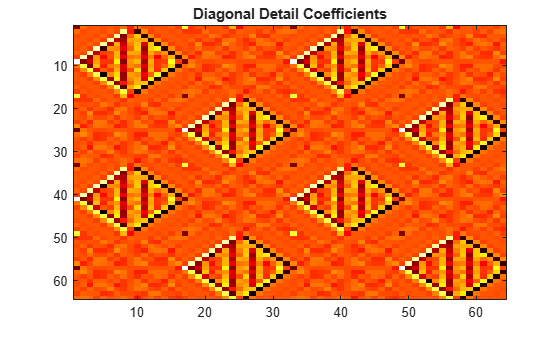```imagesc(cA) title('Approximation Coefficients')```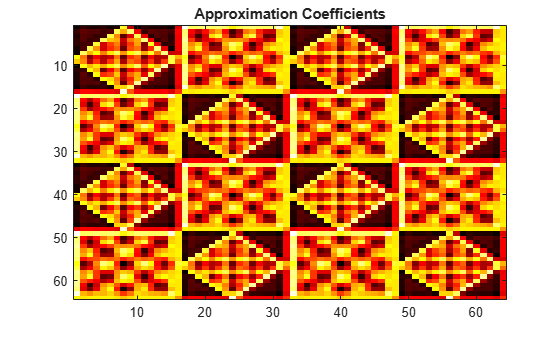```load sculpture imagesc(X) colormap gray```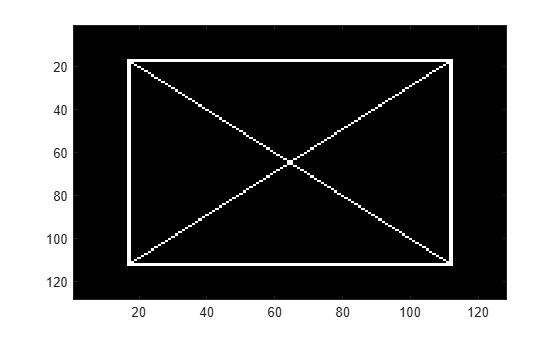Generate the lowpass and highpass decomposition filters for the Haar wavelet.

`[LoD,HiD] = wfilters('haar','d');`

Use the filters to perform a single-level 2-D wavelet decomposition. Use half-point symmetric extension. Display the approximation and detail coefficients.

```[cA,cH,cV,cD] = dwt2(X,LoD,HiD,'mode','symh'); subplot(2,2,1) imagesc(cA) colormap gray title('Approximation') subplot(2,2,2) imagesc(cH) colormap gray title('Horizontal') subplot(2,2,3) imagesc(cV) colormap gray title('Vertical') subplot(2,2,4) imagesc(cD) colormap gray title('Diagonal')```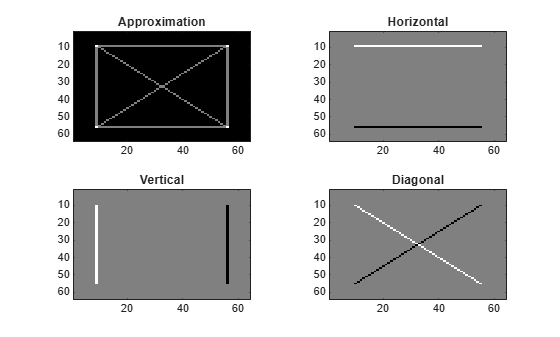Refer to GPU Computing Requirements (Parallel Computing Toolbox) to see what GPUs are supported.

Load an image. Put the image on the GPU using `gpuArray`. Save the current extension mode.

```load mask imgg = gpuArray(X); origMode = dwtmode('status','nodisp');```

Use `dwtmode` to change the extension mode to zero-padding. Obtain the single-level 2-D DWT of the image on the GPU using the `db2` wavelet.

```dwtmode('zpd','nodisp') [cA,cH,cV,cD] = dwt2(imgg,'db2');```

The current extension mode `zpd` is not supported for `gpuArray` input. Therefore, the DWT is instead performed using the `sym` extension mode. Confirm this by taking the DWT of `imgg` with the extension mode set to `sym` and compare with the previous result.

```[cAsym,cHsym,cVsym,cDsym] = dwt2(imgg,'db2','mode','sym'); [max(abs(cA(:)-cAsym(:))) max(abs(cH(:)-cHsym(:))) ... max(abs(cV(:)-cVsym(:))) max(abs(cD(:)-cDsym(:)))]```
```ans = 0 0 0 0 ```

An unsupported extension mode specified as an input argument is converted to `'sym'`. Confirm that taking the DWT of `imgg` with `'mode'` set to an unsupported mode also defaults to the `sym` extension mode.

```[cA,cH,cV,cD] = dwt2(imgg,'db2','mode','spd'); [max(abs(cA(:)-cAsym(:))) max(abs(cH(:)-cHsym(:))) ... max(abs(cV(:)-cVsym(:))) max(abs(cD(:)-cDsym(:)))]```
```ans = 0 0 0 0 ```

Change the current extension mode to periodic. Obtain the single-level DWT of the image on the GPU using the `db2` wavelet.

```dwtmode('per','nodisp') [cA,cH,cV,cD] = dwt2(imgg,'db2');```

Confirm the current extension mode `per` is supported for `gpuArray` input.

```[cAper,cHper,cVper,cDper] = dwt2(imgg,'db2','mode','per'); [max(abs(cA(:)-cAper(:))) max(abs(cH(:)-cHper(:))) ... max(abs(cV(:)-cVper(:))) max(abs(cD(:)-cDper(:)))]```
```ans = 0 0 0 0 ```

Restore the extension mode to the original setting.

`dwtmode(origMode,'nodisp')`

## Input Arguments

collapse all

Input data, specified as a numeric or logical array. `X` can be an `m`-by-`n` array representing an indexed image or an `m`-by-`n`-by-3 array representing a truecolor image. For more information on truecolor images, see RGB (Truecolor) Images.

Data Types: `double` | `single` | `uint8`

Analyzing wavelet used to compute the 2-D DWT, specified as a character vector or string scalar. The analyzing wavelet is from one of the following wavelet families: Best-localized Daubechies, Beylkin, Coiflets, Daubechies, Fejér-Korovkin, Haar, Han linear-phase moments, Morris minimum-bandwidth, Symlets, Vaidyanathan, Discrete Meyer, Biorthogonal, and Reverse Biorthogonal. See `wfilters` for the wavelets available in each family.

Data Types: `char` | `string`

Wavelet decomposition lowpass filter, specified as an even-length real-valued vector. `LoD` must be of the same length as `HiD`.

Data Types: `double` | `single`

Wavelet decomposition highpass filter, specified as an even-length real-valued vector. `HiD` must be of the same length as `LoD`.

Data Types: `double` | `single`

Extension mode used when performing the DWT, specified as one of the following:

`mode`

DWT Extension Mode

`'zpd'`

Zero extension

`'sp0'`

Smooth extension of order 0

`'spd'` (or``` 'sp1'```)

Smooth extension of order 1

`'sym'` or `'symh'`

Symmetric extension (half point): boundary value symmetric replication

`'symw'`

Symmetric extension (whole point): boundary value symmetric replication

`'asym'` or `'asymh'`

Antisymmetric extension (half point): boundary value antisymmetric replication

`'asymw'`

Antisymmetric extension (whole point): boundary value antisymmetric replication

`'ppd'`

Periodized extension (1)

`'per'`

Periodized extension (2)

If the signal length is odd, `wextend` adds to the right an extra sample that is equal to the last value, and performs the extension using the `'ppd'` mode. Otherwise, `'per'` reduces to `'ppd'`. This rule also applies to images.

The global variable managed by `dwtmode` specifies the default extension mode.

Example: ```[cA,cH,cV,cD] = dwt2(x,'db4','mode','symw');```

## Output Arguments

collapse all

Approximation coefficients, returned as an array whose size depends on `X`. Let `sx = size(X)` and `lf = `the length of the decomposition filters.

• If the DWT extension mode is set to periodization, then this output is of size `ceil(sx/2)`.

• For the other extension modes, this output is of size `floor((sx+lf-1)/2)`.

Data Types: `double`

Horizontal detail coefficients, returned as an array whose size depends on `X`. Let `sx = size(X)` and `lf = `the length of the decomposition filters.

• If the DWT extension mode is set to periodization, then this output is of size `ceil(sx/2)`.

• For the other extension modes, this output is of size `floor((sx+lf-1)/2)`.

Data Types: `double`

Vertical detail coefficients, returned as an array whose size depends on `X`. Let `sx = size(X)` and `lf = `the length of the decomposition filters.

• If the DWT extension mode is set to periodization, then this output is of size `ceil(sx/2)`.

• For the other extension modes, this output is of size `floor((sx+lf-1)/2)`.

Data Types: `double`

Diagonal detail coefficients, returned as an array whose size depends on `X`. Let `sx = size(X)` and `lf =` the length of the decomposition filters.

• If the DWT extension mode is set to periodization, then this output is of size `ceil(sx/2)`.

• For the other extension modes, this output is of size `floor((sx+lf-1)/2)`.

Data Types: `double`

## Algorithms

The 2-D wavelet decomposition algorithm for images is similar to the one-dimensional case. The two-dimensional wavelet and scaling functions are obtained by taking the tensor products of the one-dimensional wavelet and scaling functions. This kind of two-dimensional DWT leads to a decomposition of approximation coefficients at level j in four components: the approximation at level j + 1, and the details in three orientations (horizontal, vertical, and diagonal). The following chart describes the basic decomposition steps for images.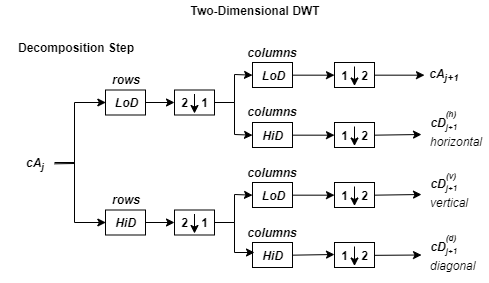where

•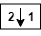— Downsample columns: keep the even-indexed columns

•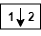— Downsample rows: keep the even-indexed rows

•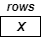— Convolve with filter X the rows of the entry

•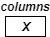— Convolve with filter X the columns of the entry

The decomposition is initialized by setting the approximation coefficients equal to the image s: cA0 = s.

Note

To deal with signal-end effects introduced by a convolution-based algorithm, the 1-D and 2-D DWT use a global variable managed by `dwtmode`. This variable defines the kind of signal extension mode used. The possible options include zero-padding and symmetric extension, which is the default mode.

 Daubechies, Ingrid. Ten Lectures on Wavelets. CBMS-NSF Regional Conference Series in Applied Mathematics 61. Philadelphia, Pa: Society for Industrial and Applied Mathematics, 1992.

 Mallat, S.G. “A Theory for Multiresolution Signal Decomposition: The Wavelet Representation.” IEEE Transactions on Pattern Analysis and Machine Intelligence 11, no. 7 (July 1989): 674–93. https://doi.org/10.1109/34.192463.

 Meyer, Y. Wavelets and Operators. Translated by D. H. Salinger. Cambridge, UK: Cambridge University Press, 1995.